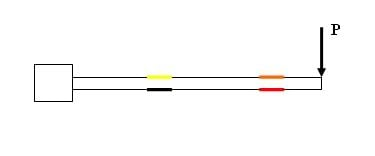# Wheatstone Bridge Strain Gauge Question

## Homework Statement

I recently did a bending lab with a WB Strain Gauge in a bunch of different configurations.

One measurement in particular is bothering me. Referring to the figure below, there are four strain gauges, Yellow, Orange, Black and Red mounted to a cantilevered beam with an applied loading 'P.'

I had the data acquisition system output a measurement for a Half-Bridge configuration in which R1 = RYellow and R2 = ROrange

We can see from the figure that both of the gauges are in tension. So why is the strain measurement that I got negative? It is effectively taking the strain measured from the yellow gauge and subtracting it from the strain ,measured from the orange gauge. Why does it do that? What exactly is this quantifying?Thanks,
Casey

Also: I thought maybe I screwed this up, but the Lab Assistant had all of the 'approximate expected strains' and mine matched up pretty well.

## Answers and Replies

Harry Hazard
Since you said your strain "number" is correct and your "sign" is wrong, my first guess is to check your resistance to strain formula.

Classically, this would be (R_strained-R_original)/(R/original*Gage Factor).

Reversing the R_original and R_strained in the numerator would produce the same result, only negative.

Since you said your strain "number" is correct and your "sign" is wrong, my first guess is to check your resistance to strain formula.

Classically, this would be (R_strained-R_original)/(R/original*Gage Factor).

Reversing the R_original and R_strained in the numerator would produce the same result, only negative.

Hey thereThanks for having a look, but just to clarify:

I never said that my sign was wrong. I just don't understand what is being measured here. Why does the half bridge take one measurement and subtract it from the other? What does that get me?

Thanks!

Harry Hazard
Oops! I misread that. :D

You measure the voltage between two opposite points, lets say "North" and "South" if your bridge has the diamond configuration. And you apply a voltage from "West" to "East". On the South bridge, you have two known resistors. On the North, you have one known resistor and one unknown resistor(the strain gage). You can solve for the unknown resistor using the known resistors and voltages. And at this point, once I get my scanner working, I'll scan in my notes on it. lol